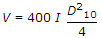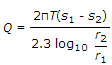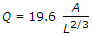Courses

# Test: Water Resources Engineering - 2

## 25 Questions MCQ Test Water Resources Engineering | Test: Water Resources Engineering - 2

Description
This mock test of Test: Water Resources Engineering - 2 for Civil Engineering (CE) helps you for every Civil Engineering (CE) entrance exam. This contains 25 Multiple Choice Questions for Civil Engineering (CE) Test: Water Resources Engineering - 2 (mcq) to study with solutions a complete question bank. The solved questions answers in this Test: Water Resources Engineering - 2 quiz give you a good mix of easy questions and tough questions. Civil Engineering (CE) students definitely take this Test: Water Resources Engineering - 2 exercise for a better result in the exam. You can find other Test: Water Resources Engineering - 2 extra questions, long questions & short questions for Civil Engineering (CE) on EduRev as well by searching above.
QUESTION: 1

Solution:
QUESTION: 2

Solution:
QUESTION: 3

### If y is the depth of water at any section, then the mean velocity is

Solution:
QUESTION: 4

The run off is affected by

Solution:
QUESTION: 5

The initial basin recharge is equal to

Solution:
QUESTION: 6

The run off a drainage basin is

Solution:
QUESTION: 7

For computing the run off volumes of large areas, number of infiltrations used are

Solution:

number of infiltrometer is 2.
1, single ring.
2, double ring.

QUESTION: 8

From the Survey of India map, the distance of the critical point is 20 km and difference in elevation is 193 m. The over land flow time, is

Solution:
QUESTION: 9

If the area of storm hydrograph is equal to 102 cm, the ordinates of a unit hydrograph may be obtained by dividing the ordinates of the storm hydrograph by

Solution:
QUESTION: 10

If s is the potential infiltration, P is rainfall in cm in a drainage of a soil with fair pasture cover, the direct run off Q in cm is given by

Solution:
QUESTION: 11

If the potential infiltration of a water shed having a soil with fair pasture cover, is 10 cm and rainfall is 12 cm, the direct run off is :

Solution:
QUESTION: 12

The quantity of water retained by the sub-soil against gravity, is known

Solution:
QUESTION: 13

Pick up the incorrect statement from the following :

Solution:
QUESTION: 14

With the usual meanings ofletters, the equationis used for determining the velocity of ground water flow in metres per day. It is known as

Solution:
QUESTION: 15

If the viscosity of ground water is 1.00, the Slitcher's constant is 400, the effective size of soil particles in acquifer is 0.5 mm and hydraulic gradient is 1 in 80, the velocity of flow is

Solution:
QUESTION: 16

In the derivation of Thiem's formula, the following assumption is not applicable

Solution:
QUESTION: 17

Pick up the correct statement from the following :

Solution:
QUESTION: 18

A well penetrates to 30 m below the static water table. After 24 hours of pumping at 31.40 litres/minute, the water level in a test well at a distance of 80 m is lowered by 0.5 m and in a well 20 m away water is lowered by 1.0 m. The transmissibility of the auifer, is

Solution:
QUESTION: 19

Shrouding is provided in

Solution:
QUESTION: 20

The efficiency of a pump may be taken as

Solution:
QUESTION: 21

For calculating the evaporation rate over a reservoir surface E = 0.771 (1.465 - 0.00732 B) (0.44 - 0.007375 V) (pe - pa), the equation is given by

Solution:
QUESTION: 22

Consumptive use of a crop during growth, is the amount of

Solution:
QUESTION: 23

For the estimate of high floods in fan-shaped catchment, the formula used is

Solution:
QUESTION: 24

Burge formulacumecs is based upon

Solution:
QUESTION: 25

Ryve's formula for flood estimate in cumecs, is

Solution: